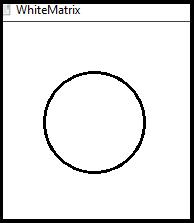# How to draw a circle in OpenCV using C++?

A circle has a center and a radius. To draw a circle using OpenCV, we have to define the center and the radius. In OpenCV we have to include <imgproc.hpp> header because 'circle()' function is defined in this header.

The basic syntax of this method is as follows −

## Syntax

circle(whiteMatrix, center,radius, line_Color, thickness);

The following program represents how to draw a circle in OpenCV.

## Example

#include<iostream>
#include<opencv2/highgui/highgui.hpp>
#include<opencv2/imgproc/imgproc.hpp>
using namespace cv;
using namespace std;
int main() {
Mat whiteMatrix(200, 200, CV_8UC3, Scalar(255, 255, 255));//Declaring a white matrix
Point center(100, 100);//Declaring the center point
Scalar line_Color(0, 0, 0);//Color of the circle
int thickness = 2;//thickens of the line
namedWindow("whiteMatrix");//Declaring a window to show the circle
circle(whiteMatrix, center,radius, line_Color, thickness);//Using circle()function to draw the line//
imshow("WhiteMatrix", whiteMatrix);//Showing the circle//
waitKey(0);//Waiting for Keystroke//
return 0;
}

## Output